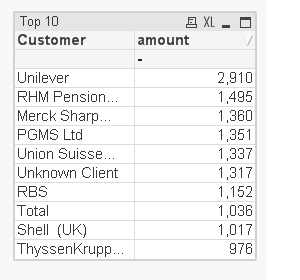# New to QlikView

Discussion board where members can get started with QlikView.

Highlighted
Contributor

## Top 10 customers, linked to variables in a slider

Good day,

Hope all is well and hoping you can help me with a quick query

Basically I have the following calculation, that enables me to create a slider using variables, where you can choose the min and max customer amounts to include/exclude.

=Num(Sum({\$<Year_YYYY={'\$(=vMaxYear)'},Customer={"=Sum(amount)<=\$(vMinLimit) or Sum(amount)>=\$(vMaxLimit)"}>} amount)/1000,'#,###0')

I now want to create another table, just to show me a top 10, as per calculation below

=Num(sum({<Year_YYYY={'\$(vMaxYear)'},Customer = {'=rank(sum(amount),4)<=10'}>} amount)/1000,'#,###0')

I would like to put this top 10 ranking calc, into the above variables calculation, but am struggling to combine the two.

I attach a qvd for refrence

Any help, would be great.

Tags (4)
1 Solution

Accepted SolutionsMVP

## Re: Top 10 customers, linked to variables in a slider

Try this expression

IF(

rank(total Sum({\$<Year_YYYY={'\$(=vMaxYear)'},Customer={"=Sum(amount)<=\$(vMinLimit) or Sum(amount)>=\$(vMaxLimit)"}>}amount)/1000)<=10,

Num(Sum({\$<Year_YYYY={'\$(=vMaxYear)'},Customer={"=Sum(amount)<=\$(vMinLimit) or Sum(amount)>=\$(vMaxLimit)"}>}amount)/1000,'#,###0')

)

6 RepliesMVP

## Re: Top 10 customers, linked to variables in a slider

Try this expression

IF(

rank(total Sum({\$<Year_YYYY={'\$(=vMaxYear)'},Customer={"=Sum(amount)<=\$(vMinLimit) or Sum(amount)>=\$(vMaxLimit)"}>}amount)/1000)<=10,

Num(Sum({\$<Year_YYYY={'\$(=vMaxYear)'},Customer={"=Sum(amount)<=\$(vMinLimit) or Sum(amount)>=\$(vMaxLimit)"}>}amount)/1000,'#,###0')

)

Contributor

## Re: Top 10 customers, linked to variables in a slider

Hi Manish,

Many thanks for taking the time, to giving me some adviceI like the calc, but do you know why this removes the total amount, as you can see below?

ThanksMVP

## Re: Top 10 customers, linked to variables in a slider

Try

SUM(Aggr(IF(

rank(total Sum({\$<Year_YYYY={'\$(=vMaxYear)'},Customer={"=Sum(amount)<=\$(vMinLimit) or Sum(amount)>=\$(vMaxLimit)"}>}amount)/1000)<=10,

Num(Sum({\$<Year_YYYY={'\$(=vMaxYear)'},Customer={"=Sum(amount)<=\$(vMinLimit) or Sum(amount)>=\$(vMaxLimit)"}>}amount)/1000,'#,###0')

),Period_Sort,Period_Number,Month))MVP

## Re: Top 10 customers, linked to variables in a slider

Or if this is a straight table just use Sum of rows on the expressions tab

Contributor

## Re: Top 10 customers, linked to variables in a slider

ahhh yes, something so simple yet leaves the brain sometimes haha

many thanks Sunny, very helpful as ever

Contributor

## Re: Top 10 customers, linked to variables in a slider

this was the pefect calc, appreciate the response, its very much appreciated.

Added into the sum expression from Sunny, it's all working

have a good weekend both